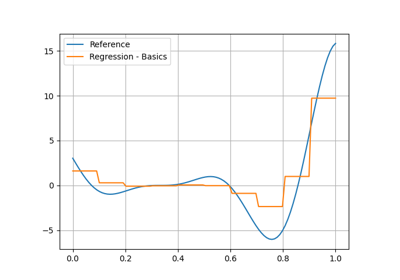gemseo / mlearning / regression

# random_forest module¶

Random forest regression model.

Use an ensemble of decision trees.

## Dependence¶

The regression model relies on the RandomForestRegressor class of the scikit-learn library.

class gemseo.mlearning.regression.random_forest.RandomForestRegressor(data, transformer=mappingproxy({}), input_names=None, output_names=None, n_estimators=100, **parameters)[source]

Random forest regression.

Parameters:
• data (IODataset) – The learning dataset.

• transformer (TransformerType) –

The strategies to transform the variables. The values are instances of Transformer while the keys are the names of either the variables or the groups of variables, e.g. "inputs" or "outputs" in the case of the regression algorithms. If a group is specified, the Transformer will be applied to all the variables of this group. If IDENTITY, do not transform the variables.

By default it is set to {}.

• input_names (Iterable[str] | None) – The names of the input variables. If None, consider all the input variables of the learning dataset.

• output_names (Iterable[str] | None) – The names of the output variables. If None, consider all the output variables of the learning dataset.

• n_estimators (int) –

The number of trees in the forest.

By default it is set to 100.

• **parameters – The parameters of the machine learning algorithm.

Raises:

ValueError – When both the variable and the group it belongs to have a transformer.

LIBRARY: Final[str] = 'scikit-learn'

The name of the library of the wrapped machine learning algorithm.

SHORT_ALGO_NAME: ClassVar[str] = 'RF'

The short name of the machine learning algorithm, often an acronym.

Typically used for composite names, e.g. f"{algo.SHORT_ALGO_NAME}_{dataset.name}" or f"{algo.SHORT_ALGO_NAME}_{discipline.name}".

algo: Any

The interfaced machine learning algorithm.

input_names: list[str]

The names of the input variables.

input_space_center: dict[str, ndarray]

The center of the input space.

learning_set: Dataset

The learning dataset.

output_names: list[str]

The names of the output variables.

parameters: dict[str, MLAlgoParameterType]

The parameters of the machine learning algorithm.

transformer: dict[str, Transformer]

The strategies to transform the variables, if any.

The values are instances of Transformer while the keys are the names of either the variables or the groups of variables, e.g. “inputs” or “outputs” in the case of the regression algorithms. If a group is specified, the Transformer will be applied to all the variables of this group.

## Examples using RandomForestRegressor¶Quality measure for surrogate model comparison

Quality measure for surrogate model comparisonRandom forest regression

Random forest regression